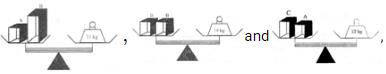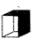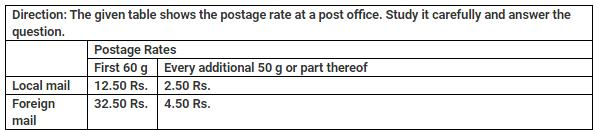Courses

# Test : Measurement Length, Weight And Capacity

## 10 Questions MCQ Test Mathematics for Class 5 (V) - CBSE and NCERT Curriculum | Test : Measurement Length, Weight And Capacity

Description
This mock test of Test : Measurement Length, Weight And Capacity for Class 5 helps you for every Class 5 entrance exam. This contains 10 Multiple Choice Questions for Class 5 Test : Measurement Length, Weight And Capacity (mcq) to study with solutions a complete question bank. The solved questions answers in this Test : Measurement Length, Weight And Capacity quiz give you a good mix of easy questions and tough questions. Class 5 students definitely take this Test : Measurement Length, Weight And Capacity exercise for a better result in the exam. You can find other Test : Measurement Length, Weight And Capacity extra questions, long questions & short questions for Class 5 on EduRev as well by searching above.
QUESTION: 1

### 1560 ml is equal to

Solution:

we know 1000 ml = 1 L

so 1 ml = 1/1000 L

and 1560 ml = 1560 x 1/1000 = 1.560 liter

or 1 liter 560 ml

QUESTION: 2

Solution:

Not Available

QUESTION: 3

### If, then what is the mas of?

Solution:

Not Available

QUESTION: 4

Instrument used to measure the Blood Pressure is_____.

Solution:

Not Available

QUESTION: 5How much postage is needed to send a local mail of weight 310 g?

Solution:

12.50+5×2.5=Rs.25

QUESTION: 6The postage for sending a parcel by foreign mail is Rs.136. What is the maximum possible weight of the parcel?

Solution:

Not Available

QUESTION: 7

Tank A contains 6 times as much water as Tank B. How much water must Mohan transfer from Tank A to Tank B so that each tank contains 70 litres of water?

Solution:

Not Available

QUESTION: 8

Soni can cycle from point A to point B and return to point A in 10 minutes. She can cycle and walk back in 18 minutes. It will take ___ mins for her to walk there and walk back.

Solution:

Let time for cycling =x and time for walking =y We havex+x=10,x+y=18 2x=10 x=5
∴y=13 Hence time in walking from both sides=26 minutes

QUESTION: 9

Rajesh bought 13 kg of peanuts. He saved? 195 by using a store coupon. How much did he save per kg of ground nuts?

Solution:

Saving per kg =195/13=15

QUESTION: 10

Julie has Rs. 61.50 to buy lunch. If she buys a burger that costs Rs. 42.75, how much money is left with her?

Solution:

Money left with Julie  =61.50−42.75=18.75